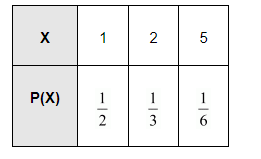# The mean of the numbers obtained on throwing a die having written 1 on three faces, 2 on two faces and 5 on one face is`
Question:

The mean of the numbers obtained on throwing a die having written 1 on three faces, 2 on two faces and 5 on one face is

(A) 1

(B) 2

(C) 5

(D) $\frac{8}{3}$

Solution:

Let X be the random variable representing a number on the die.

The total number of observations is six.

$\therefore P(X=1)=\frac{3}{6}=\frac{1}{2}$

$\mathrm{P}(\mathrm{X}=2)=\frac{2}{6}=\frac{1}{3}$

$\mathrm{P}(\mathrm{X}=5)=\frac{1}{6}$

Therefore, the probability distribution is as follows.Mean $=\mathrm{E}(\mathrm{X})=\sum p_{i} x_{i}$

$=\frac{1}{2} \times 1+\frac{1}{3} \times 2+\frac{1}{6} \cdot 5$

$=\frac{1}{2}+\frac{2}{3}+\frac{5}{6}$

$=\frac{3+4+5}{6}$

$=\frac{12}{6}$

$=2$